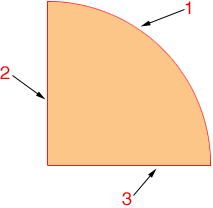SEARCH HOMEMath Central Quandaries & QueriesQuestion from kim, a student: how do you figure the perimeter of a quarter circle that has a radius of 7 inches? I would like to have the explanation of how you figure it out. Thank youHi Kim,

The perimeter of a quarter is made up of 3 parts.The first part is of length one-quarter of the perimeter (circumference) of the circle.

What is the length of one-quarter the circumference of a circle of radius 7 inches?

Parts 2 and 3 are each a radius of the circle.

What is the sum of the lengths of the three parts?

PennyMath Central is supported by the University of Regina and The Pacific Institute for the Mathematical Sciences.